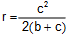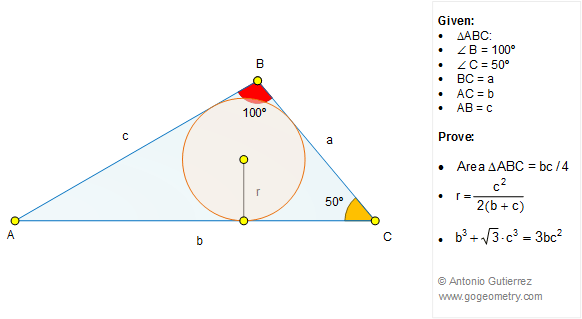# Geometry Problem 1288: Unlocking the Geometry of Triangles: Exploring 30-50-100 Degrees, Area, Inradius, and Metric Relations

In triangle ABC, where angle B is 100 degrees, angle C is 50 degrees, and the lengths of sides BC, AC, and AB are denoted by a, b, and c respectively. Prove that: (1) the area of triangle ABC = bc/4; (2) Inradius; (3).
This entry contributed by Sumith Peiris, Moratuwa, Sri Lanka.